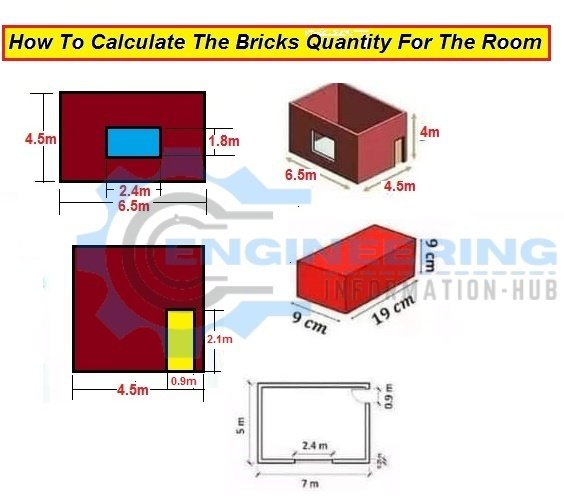# How To Calculate The Bricks Quantity For Room

In This Article, I will learn how to calculate the bricks for the room| Calculate The Bricks For Room | how to calculate the bricks quantity for the room. this is a basic and very important topic related to the civil engineer, site supervisor, and quantity surveyor.

we have some given data in the below image we will find the number of bricks with the help of the given data.### given data

length of the room =6.5m

width of the room = 4.5m

Height of the room =4m

The thickness of all walls = 0.25m

### Solution

size of the door= L x H

size of the door = 2.1 x 0.9

volume of the  door portion= 2.1 x 0.9 x 0.25=0.4725m³

size of the window=L x H

size of the window= 2.4 x 1.8=4.32m²

The volume of the window portion = 2.4 x 1.8 x 0.25 =1.08m³

size of the bricks = 19cm x 9cm x 9cm

length of long wall =L x Number of wall

length of long wall = 6 x 2 =12m

volume of long wall= L x H x t

### How To Calculate The Quantity of Cement Mortar In Brickwork And Plaster

volume of long wall=12x 4 x 0.25 =12m³

length of short wall =L x Number of wall

length of short wall = 4.5 x 2=9m

volume of short wall =  L x H x t

volume of short wall = 9x 4 x 0.25=9m²

Deduction of the window from long wall = volume of a long wall – the volume of a window

Deduction of window from long wall = 12 -1.08 = 10.92m²

Deduction of the Door from the short wall = volume of a short wall – the volume of the door

Deduction of the Door from the short wall = 9 – 0.472 = 8.953m²

The total volume of the room = volume of the long wall without a window +volume of a short wall without a window

The total volume of the room =10.92+8.953 = 19.873m³

Thickness of motor =10mm =0.01m

Volume of bricks with motor =0.20cm x0.10cm x0.10cm = 0.002m³

Total Number of Bricks = Total Volume of room / Volume of bricks with motor

Total Number of Bricks =19.873/ 0.002

Total Number of Bricks =9936.4 = 9937bricks

Add 5% extra wastage of bricks = 9937/100 x 5 = 496.85 =497bricks

Total Number of bricks = 9937+497  = 10434 bricks

I hope you learn better. if you have any questions feel free to give the comments I will appreciate your question. get benefits and share with others.